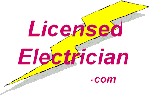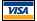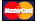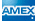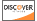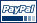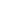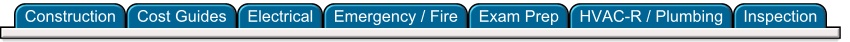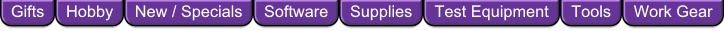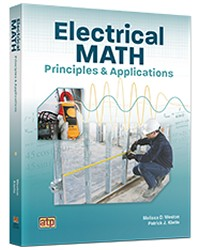Electrical Math Principles and Applications AT-1849 \$68.00Related: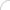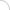Save \$\$: ElectriCalc Pro NEC Calculator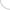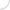# Electrical Math Principles and Applications

By: Melissa D. Weston, Patrick J. Klette

## Electrical Math Principles and Applications presents math principles in an electrical context so that learners understand their relevance.

Electrical Math Principles and Applications demonstrates how electrical technicians use math on the job. From the fundamentals to advanced math concepts, this text/workbook presents math principles in an electrical context so that learners understand their relevance.

Each chapter is divided into smaller sections that present basic math principles in arithmetic, notations, numbering systems, measurements, conversions, formulas, equations, percentages, ratios, graphs, trigonometry, vectors, and complex numbers.

Features:

• Electrical applications provide step-by-step explanations and graphics showcasing how math is used to solve problems in the field.
• Checkpoint questions at the end of each section assess comprehension of math principles.
Math exercises at the end of each chapter ask learners to use math principles to solve electrical problems.
• Complimentary online learner resources provide additional opportunities to learn and apply math skills.

Contents include:

Whole Numbers, Integers, and Decimals in the Electrical Field

• Whole Numbers
• Integers
• Decimals

Fractions and Mixed Numbers in the Electrical Field

• Fractions and Mixed Numbers
• Working with Fractions

Notations and Numbering Systems in the Electrical Field

• Standard and Scientific Notation
• Engineering Notation and Prefixes
• Alternative Numbering Systems

Electrical Measurement Systems and Conversions

• US Customary System and Metric System Conversions
• Conversions Between Measurement Systems
• Accuracy and Precision

Electrical Formulas and Equations

• Performing Operations in Electrical Formulas
• Solving Electrical Equations and Formulas
• Applying Electrical Formulas

Electrical Applications of Percentages and Ratios

• Fractions, Decimals, and Percentages
• Ratios, Rates, and Proportions
• Percent Increases and Decreases
• Direct and Inverse Variation

Electrical Equations and Graphs

• Interpreting and Creating Graphs
• Graphing Equations

Electrical Applications of Right Triangle Trigonometry

• Right Triangles and Pythagorean Theorem
• Trigonometric Ratios

Electrical Applications of Sinusoidal Trigonometry

• Sine Waves
• Instantaneous and Peak Values
• Common AC Amplitudes

Electrical Predictions Using Vectors

• Vector Magnitude and Vector Direction
• Horizontal and Vertical Vector Components
• Law of Sines and Law of Cosines

Electrical Applications of Complex Numbers

• Expressing Complex Numbers
• Basic Operations with Complex Numbers

Softcover
 Related: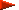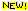Electrical EstimatingCost Guides & Cost DataIndustrial ElectricityPLCs & Motor ControlsElectrical Exam PrepElectrical TheoryElectrical CalculationsElectrical SuppliesTools for Electricians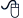Order online: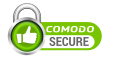Add the item(s) to your shopping cart and submit your order online through an encrypted secure server. Major Credit Cards and PayPal are accepted.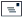Order offline: Print an offline order form and Mail or Fax your order to us.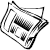Subscribe to Our Newsletter for New Product info, Announcements & Specials Contact us:  Store@LicensedElectrician.comBlue Print Reading   Business/Office    Calculators/Measuring   Inspection   Insulated-Tools   Legal   Low-Voltage   Maintenance   Motor Control / PLC    New / Specials    Plumbing   PPE    Rack-A-Tiers   Safety    Small-Engines    SmartBox[ Home ] [ Contents ] [ Feedback ] [ Search ] [ News ]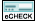LicensedElectrician.com